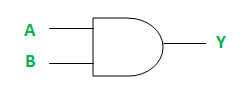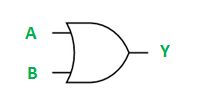GeeksforGeeks App
Open AppBrowser
Continue

# VHDL code for AND and OR Logic Gates

Prerequisite – Introduction of Logic Gates

Design and implement the AND and OR logic gates using VHDL (VHSIC Hardware Description Language) programming language.

Different Types of VHDL Modelling Styles

The architecture of VHDL code is written in three different coding styles :

1. Dataflow Modelling
2. Behavioral Modelling
3. Structural Modelling

1. Logic Development for AND Gate: The AND logic gate can be realized as follows –The truth table for AND Gate is:

Implementation of Dataflow Modelling – Below is the implementation of the above logic in the VHDL language (Dataflow Modelling).

```-- VHDL Code for AND gate

library IEEE;
use IEEE.std_logic_1164.all;

-- Entity declaration

entity andGate is

port(A : in std_logic;      -- AND gate input
B : in std_logic;      -- AND gate input
Y : out std_logic);    -- AND gate output

end andGate;

-- Dataflow Modelling Style
-- Architecture definition

architecture andLogic of andGate is

begin

Y <= A AND B;

end andLogic; ```

2. Logic Development for OR Gate: The OR logic gate can be realized as follows –The truth table for OR Gate is:

Implementation of Dataflow Modelling – Below is the implementation of the above logic in the VHDL language (Dataflow Modelling).

```-- VHDL Code for OR gate

library IEEE;
use IEEE.std_logic_1164.all;

-- Entity declaration

entity orGate is

port(A : in std_logic;      -- OR gate input
B : in std_logic;      -- OR gate input
Y : out std_logic);    -- OR gate output

end orGate;

-- Dataflow Modelling Style
-- Architecture definition

architecture orLogic of orGate is

begin

Y <= A OR B;

end orLogic;```
My Personal Notes arrow_drop_up